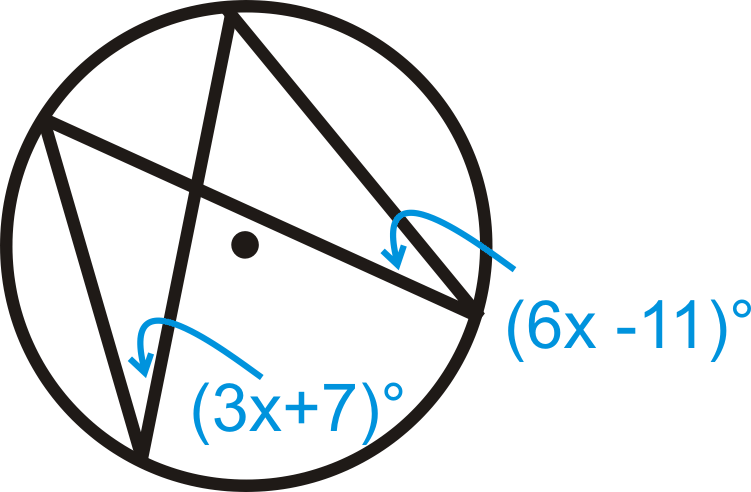# 6.14: Inscribed Angles in Circles

•• Contributed by CK12
• CK12

Vertex on a circle and chords as sides, and whose measure equals half the intercepted arc.

An inscribed angle is an angle with its vertex on the circle and whose sides are chords. The intercepted arc is the arc that is inside the inscribed angle and whose endpoints are on the angle. The vertex of an inscribed angle can be anywhere on the circle as long as its sides intersect the circle to form an intercepted arc.Figure $$\PageIndex{1}$$

The Inscribed Angle Theorem states that the measure of an inscribed angle is half the measure of its intercepted arc.Figure $$\PageIndex{2}$$

$$m\angle ADC=\dfrac{1}{2}m\widehat{AC}$$ and $$m\widehat{AC}=2m\angle ADC$$

Inscribed angles that intercept the same arc are congruent. This is called the Congruent Inscribed Angles Theorem and is shown below.Figure $$\PageIndex{3}$$

$$\angle ADB$$ and $$\angle ACB$$ intercept $$\widehat{AB}$$, so $$m\angle ADB=m\angle ACB$$. Similarly, $$\angle DAC$$ and $$\angle DBC$$ intercept $$\widehat{DC}$$, so $$m\angle DAC=m\angle DBC$$.

An angle intercepts a semicircle if and only if it is a right angle (Semicircle Theorem). Anytime a right angle is inscribed in a circle, the endpoints of the angle are the endpoints of a diameter and the diameter is the hypotenuse.

What if you had a circle with two chords that share a common endpoint? How could you use the arc formed by those chords to determine the measure of the angle those chords make inside the circle?

Example $$\PageIndex{1}$$

Find $$m\widehat{DC}$$ and $$m\angle ADB$$.Figure $$\PageIndex{4}$$

Solution

From the Inscribed Angle Theorem:

\begin{aligned} m\widehat{DC}&=2\cdot 45^{\circ}=90^{\circ} \\ m\angle ADB&=12\cdot 76^{\circ}=38^{\circ}\end{aligned}

Example $$\PageIndex{2}$$

Find $$m\angle ADB$$ and $$m\angle ACB$$.Figure $$\PageIndex{5}$$

Solution

The intercepted arc for both angles is $$\widehat{AB}$$. Therefore,

\begin{aligned} m\angle ADB&=12\cdot 124^{\circ}=62^{\circ} \\ m\angle ACB&=12\cdot 124^{\circ}=62^{\circ}\end{aligned}

Example $$\PageIndex{3}$$

Find $$m\angle DAB$$ in $$\bigodot C$$.Figure $$\PageIndex{6}$$

Solution

C is the center, so $$\overline{DB}$$ is a diameter. $$\angle DAB$$'s endpoints are on the diameter, so the central angle is $$180^{\circ}$$.

$$m\angle DAB=\dfrac{1}{2}\cdot 180^{\circ}=90^{\circ}$$.

Example $$\PageIndex{4}$$

Fill in the blank: An inscribed angle is ____________ the measure of the intercepted arc.

Solution

half

Example $$\PageIndex{5}$$

Fill in the blank: A central angle is ________________ the measure of the intercepted arc.

Solution

equal to

## Review

Fill in the blanks.

1. An angle inscribed in a ________________ is $$90^{\circ}$$.
2. Two inscribed angles that intercept the same arc are _______________.
3. The sides of an inscribed angle are ___________________.
4. Draw inscribed angle $$\angle JKL$$ in $$\bigodot M$$. Then draw central angle $$\angle JML$$. How do the two angles relate?

Find the value of $$x$$ and/or $$y$$ in $$\bigodot A$$.

1.Figure $$\PageIndex{7}$$
2.Figure $$\PageIndex{8}$$
3.Figure $$\PageIndex{9}$$
4.Figure $$\PageIndex{10}$$
5.Figure $$\PageIndex{11}$$

Solve for $$x$$.

1.Figure $$\PageIndex{12}$$
2.Figure $$\PageIndex{13}$$
3.Figure $$\PageIndex{14}$$
4.Figure $$\PageIndex{15}$$
5. Fill in the blanks of the Inscribed Angle Theorem proof.Figure $$\PageIndex{16}$$

Given: Inscribed $$\angle ABC$$ and diameter $$\overline{BD}$$

Prove: $$m\angle ABC=12m\widehat{AC} Statement Reason 1. Inscribed \(\angle ABC$$ and diameter $$\overline{BD}$$

$$m\angle ABE=x^{\circ}$$ and $$m\angle CBE=y^{\circ}$$

1.
2. $$x^{\circ}+y^{\circ}=m\angle ABC$$ 2.
3. 3. All radii are congruent
4. 4. Definition of an isosceles triangle
5. $$m\angle EAB=x^{\circ}$$ and $$m\angle ECB=y^{\circ}$$ 5.
6. $$m\angle AED=2x^{\circ}$$ and $$m\angle CED=2y^{\circ}$$ 6.
7. $$m\widehat{AD}=2x^{\circ}$$ and $$m\widehat{DC}=2y^{\circ}$$ 7.
9. $$m\widehat{AC}=2x^{\circ}+2y^{\circ}$$ 9.
10. 10. Distributive PoE
11. $$m\widehat{AC}=2m\angle ABC$$ 11.
12. $$m\angle ABC=\dfrac{1}{2}m\widehat{AC}$$ 12.

## Vocabulary

Term Definition
central angle An angle formed by two radii and whose vertex is at the center of the circle.
chord A line segment whose endpoints are on a circle.
circle The set of all points that are the same distance away from a specific point, called the center.
diameter A chord that passes through the center of the circle. The length of a diameter is two times the length of a radius.
Inscribed Angle An inscribed angle is an angle with its vertex on the circle. The measure of an inscribed angle is half the measure of its intercepted arc.
intercepted arc The arc that is inside an inscribed angle and whose endpoints are on the angle.
radius The distance from the center to the outer rim of a circle.
Arc An arc is a section of the circumference of a circle.
Intercepts The intercepts of a curve are the locations where the curve intersects the x and y axes. An x intercept is a point at which the curve intersects the x-axis. A y intercept is a point at which the curve intersects the y-axis.
Inscribed Angle Theorem The Inscribed Angle Theorem states that the measure of an inscribed angle is half the measure of its intercepted arc.
Semicircle Theorem The Semicircle Theorem states that any time a right angle is inscribed in a circle, the endpoints of the angle are the endpoints of a diameter and the diameter is the hypotenuse.